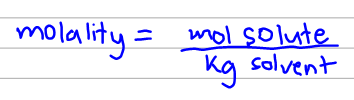# Problem: A 13.0% solution of K2CO3 by mass has a density of 1.09 g/cm3. Calculate the molality of the solution.

###### FREE Expert Solution

The molality of the solution can be calculated with the equation below:We don't have either of the values required to solve so we have to derive those first from the given values.

90% (190 ratings)###### Problem Details

A 13.0% solution of K2CO3 by mass has a density of 1.09 g/cm3. Calculate the molality of the solution.